# NCERT Solutions For Class 10 Maths Chapter 6 Exercise 6.3

## Chapter 6 Ex.6.3 Question 1

State which pairs of triangles in Figure are similar. Write the similarity criterion used by you for answering the question and also write the pairs of similar triangles in the symbolic form:

1)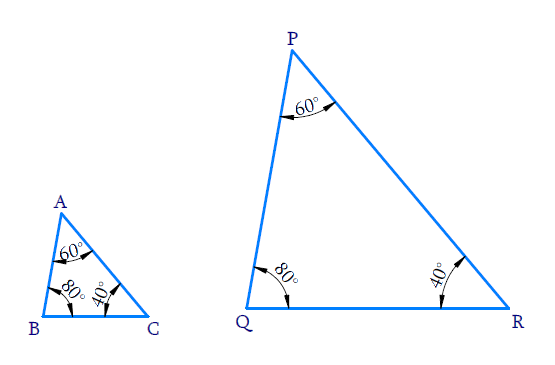2)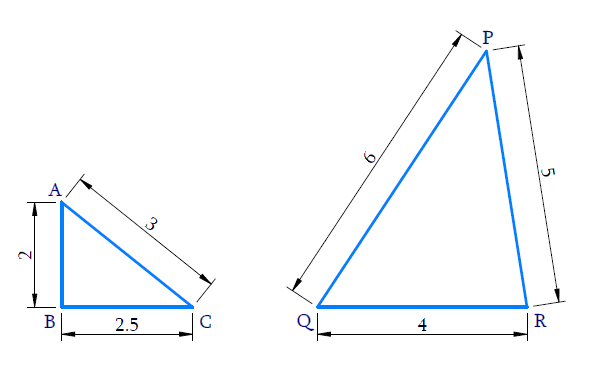3)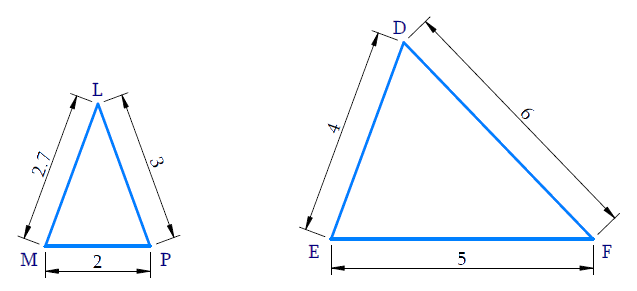4)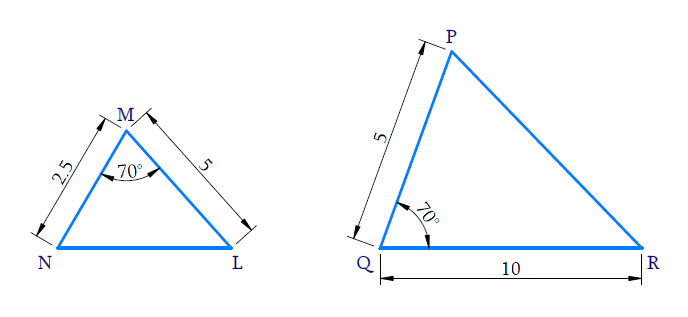5)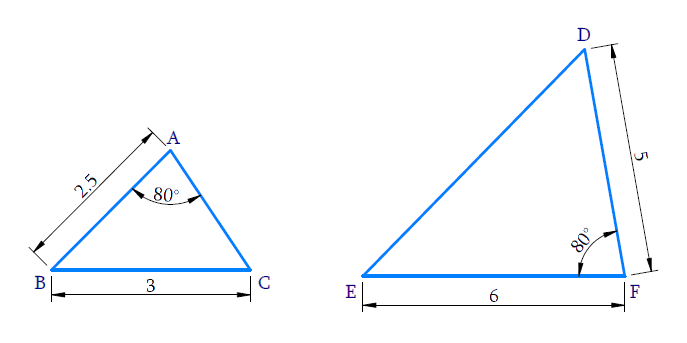6)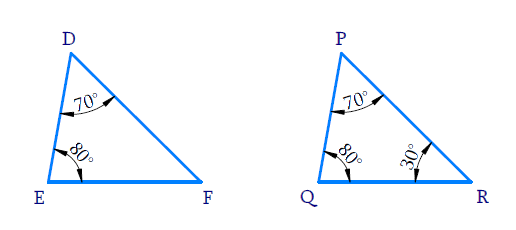### Solution

Reasoning:

As we know if in two triangles, corresponding angles are equal, then their corresponding sides are in the same ratio (or proportion) and hence the two triangles are similar.

This is referred as $$AAA$$ (Angle–Angle–Angle) criterion of similarity of two triangles.

Steps:

In $$\Delta ABC$$ and $$\Delta PQR$$

\begin{align} \angle A = \angle P = 60{}^ \circ \\ \angle B = \angle Q = {80^ \circ } \\ \angle C = \angle R = {40^ \circ } \\ \end{align}

All the corresponding angles of the triangles are equal.

By $$AAA$$ criterion \begin{align}\Delta ABC \sim \Delta PQR\end{align}

2)Reasoning :

As we know if in two triangles, side of one triangle are proportional to (i.e., in the same ratio ) the sides of the other triangle, then their corresponding angles are equal and hence the two triangles are similar.

This is referred as $$SSS$$ (Side–Side–Side) similarity criterion for two triangles.

Steps:

$$\rm{ In} \Delta ABC\, {\rm{and}}\,\Delta QRP$$

\begin{align} \frac{{AB}}{{QR}} &= \frac{2}{4} = \frac{1}{2} \\ \frac{{BC}}{{PR}} &= \frac{{2.5}}{5} = \frac{1}{2} \\ \frac{{AC}}{{PQ}} &= \frac{3}{6} = \frac{1}{2} \ \\ \Rightarrow \frac{{AB}}{{QR}} &= \frac{{BC}}{{PR}} = \frac{{AC}}{{PQ}} = \frac{1}{2} \\ \end{align}

All the corresponding sides of two triangle are in same proportion.

By $$SSS$$ criterion $$\Delta ABC \sim \Delta QPR$$

3)Reasoning:

As we know if in two triangles, side of one triangle are proportional to (i.e., in the same ratio ) the sides of the other triangle, then their corresponding angles are equal and hence the two triangles are similar.

This is referred as $$SSS$$ (Side–Side–Side) similarity criterion for two triangles.

Steps:

In $$\Delta LMP$$ and  $$\Delta FED$$

\begin{align}\frac{LM}{FE}& =\frac{2.7}{5} \\ \frac{MP}{ED}& =\frac{2}{4}=\frac{1}{2} \\ \frac{LP}{FD}&=\frac{3}{6}=\frac{1}{2} \\ \Rightarrow \frac{LM}{FE} &\ne \frac{MP}{ED}\,\,=\,\frac{LP}{FD} \end{align}

All the corresponding sides of the two triangles are not in the same proportion.

Hence triangles are not similar.

$$\Delta LMP \nsim \Delta FED$$

4)Reasoning:

As we know if one angle of a triangle is equal to one angle of the other triangle and the sides including these angles are proportional, then the two triangles are similar.

This criterion is referred to as the $$SAS$$ (Side–Angle–Side) similarity criterion for two triangles.

Steps:

In $$\Delta NML$$ and $$\Delta PQR$$

\begin{align} & \,\,\,\,\,\,\,\,\frac{NM}{PQ}=\frac{2.5}{5}=\frac{1}{2} \\ & \,\,\,\,\,\,\,\,\,\,\frac{ML}{QR}=\frac{5}{10}=\frac{1}{2} \\ & \Rightarrow \frac{NM}{PQ}=\frac{ML}{QR}=\frac{1}{2} \\ & \,\,\,\,\,\,\,\;\;\;\,\angle M=\angle Q={{70}^{\circ}} \\ \end{align}

One angle of a triangle is equal to one angle of the other triangle and the sides including these angles are proportional.

By $$SAS$$ criterion $$\Rightarrow \,\,\,\Delta NML\sim \Delta PQR$$

5)Reasoning:

As we know if one angle of a triangle is equal to one angle of the other triangle and the sides including these angles are proportional, then the two triangles are similar.

This criterion is referred to as the $$SAS$$ (Side–Angle–Side) similarity criterion for two triangles.

Steps:

In $$\Delta A B C,$$and $$\Delta D F E$$

\begin{align} & \frac{AB}{DF}=\frac{2.5}{5}=\frac{1}{2} \\ & \frac{BC}{EF}=\frac{3}{6}=\frac{1}{2} \\ & \frac{AB}{DF}=\frac{BC}{EF}=\frac{1}{2} \end{align}

$\angle A=\angle F={{80}^{\circ }}$

But $$\angle B$$ must be equal to $${{80}^{0}}$$

$$\because$$ The sides $$AB, \,BC$$ includes $$\angle B$$, not $$\angle A$$

Therefore, $$SAS$$ criterion is not satisfied

Hence,the triangles are not similar,$$\Delta ABC \nsim \Delta DFE$$

6)Reasoning:

As we know if one angle of a triangle is equal to one angle of the other triangle and the sides including these angles are proportional, then the two triangles are similar.

This criterion is referred to as the $$AAA$$ (Angle–Angle–Angle) criterion of similarity of two triangles

Steps:

In $$\Delta DEF$$

$\angle D={{70}^{0}};\angle E={{80}^{0}}$

$$\Rightarrow \angle F={{30}^{0}}$$

$$[ \because$$ Sum of the angles in a triangle is $${{180}^{0}}$$] )

Similarly,

In $$\Delta PQR$$

$\angle Q={{80}^{\circ }};\,\,\,\,\,\angle R={{30}^{\circ }}\,\,\\ \Rightarrow \angle P={{70}^{\circ }}$

In $$\Delta \,DEF\,\rm{and}\,\Delta \,PQR$$

\begin{align} & \angle D=\angle P=70{}^{0} \\ & \angle E=\angle Q={{80}^{0}} \\ & \angle F=\angle R={{30}^{0}} \\ \end{align}

All the corresponding angles of the triangles are equal.

By $$AAA$$ criterion

$$\Rightarrow \,\,\Delta DEF\sim \Delta PQR$$

Alternate method:

Reasoning:

As we are ware if two angles of one triangle are respectively equal to two angles of another triangle, then the two triangles are similar.

This may be referred to as the $$AA$$  criterion for two triangles.

Steps:

In $$\Delta DEF$$

$\angle D={{70}^{0}};\,\,\angle E={{80}^{0}}$

$$\Rightarrow \angle F={{30}^{0}}$$

$$[\because$$ Sum of the angles in a triangle is $${{180}^{\circ}}]$$

Now ,In $$\,\Delta DEF \,\rm{and}\, \Delta PQR$$

\begin{align}{\angle E}&={\angle Q=80^{\circ}} \\ {\angle F}&={\angle R=30^{\circ}} \end{align}

Pair of corresponding angles of the triangles are equal.

By $$AA$$ criterion $$\,\Delta DEF \,\sim\, \Delta PQR$$

## Chapter 6 Ex.6.3 Question 2

In Figure  $$\Delta ODC\sim \Delta OBA$$ ,$$\angle BOC = 125°$$and $$\angle CDO = 70°$$. Find ,$$\angle \text DCO, \angle \text{ DCO}$$ and $$OAB$$.

Diagram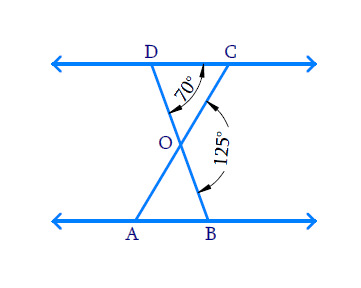### Solution

Reasoning:

As we are aware if two angles of one triangle are respectively equal to two angles of another triangle, then the two triangles are similar.

This is referred as $$AA$$ criterion for two triangles.

Steps:

In the given figure.

\begin{align} & \angle DOC={{180}^{{}^\circ }}-\angle COB \\& \left[ \begin{array} & \because \angle DOC\text{ and }\angle \text{COB}\\ \text{form a linear pair} \\\end{array} \right] \\\\& \angle DOC={{180}^{{}^\circ }}-{{125}^{{}^\circ }} \\& \angle DOC={{55}^{{}^\circ }} \\\end{align}

In $$\Delta ODC$$

\begin{align}\angle DCO&={{180}^{\circ }}-(\angle DOC+\angle ODC) \\ &[\because \,\text{angle sum property}] \\\\ \angle DCO&={{180}^{\circ }}-({{55}^{\circ }}+{{70}^{\circ }}) \\ \angle DCO &={{55}^{\circ }} \\ \end{align}

In $$\Delta ODC$$ and $$\Delta OBA$$

\begin{align} &\Delta ODC\sim \Delta OBA \\ &\quad\Rightarrow \angle DCO=\angle OAB \\ &\qquad\quad\;\; \angle DCO={{55}^{\circ }} \\ \end{align}

## Chapter 6 Ex.6.3 Question 3

Diagonals $$AC$$ and $$BD$$ of a trapezium $$ABCD$$ with $$AB\, ||\, DC$$ intersect each other at the point $$O.$$ Using a similarity criterion for two triangles, show that \begin{align}\frac{OA}{OC}=\frac{OB}{OD}\end{align}.

Diagram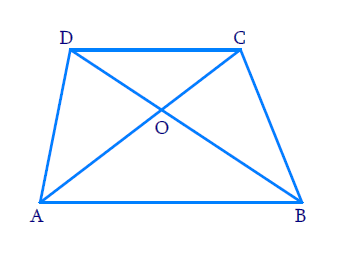### Solution

Reasoning:

If two angles of one triangle are respectively equal to the two angles of another triangle, then the two triangles are similar.

This is referred to as the $$AA$$ criterion.

Steps:

In $$\Delta \rm{A O B}, \Delta \rm{C O D}$$

\begin{align} & \angle AOB=\angle COD \\ & \text{(Vertically}\,\text{opposite}\,\,\text{angles)}\\ \\ & \angle BAO=\angle DCO \\ & (\text{Alternate interior angles }) \\\\ & \Rightarrow \Delta AOB\sim\Delta COD \\ & (\text{AA criterion}) \\ \end{align}

Hence \begin{align} \frac{O A}{O C}=\frac{O B}{O D}\end{align}

## Chapter 6 Ex.6.3 Question 4

In Figure \begin{align}\frac{QR}{QS}=\frac{QT}{PR}\end{align} and $$\angle 1 = \angle 2$$. Show that $$\Delta PQS\sim{\ }\Delta TQR$$ .

Diagram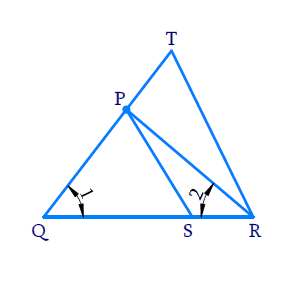### Solution

Reasoning:

As we know if one angle of a triangle is equal to one angle of the other triangle and the sides including these angles are proportional, then the two triangles are similar.

This criterion is referred to as the SAS (Side–Angle–Side) similarity criterion for two triangles.

Steps:

In $$\triangle PQR$$

\begin{align} \angle 1&=\angle 2\\ \Rightarrow \quad PR &= PQ \end{align}

(In a triangle sides opposite to equal angles are equal)

In $$\Delta PQR$$ and $$\Delta TQR$$

\begin{align} & \angle PQS=\angle TQR=\angle 1\\&(\text{Same angle}) \\\\ & \frac{QR}{QS}=\frac{QT}{PQ}\quad\left( \because \ {PR =PQ} \right) \\ & \Rightarrow \Delta PQR-\Delta TQR\\&( \because \text{ SAS criterion}) \\ \end{align}

## Chapter 6 Ex.6.3 Question 5

$$S$$ and $$T$$ are points on sides $$PR$$ and $$QR$$ of  $$\Delta PQR$$ such that  $$\angle P{\rm{ }} = \angle RTS$$. Show that

Diagram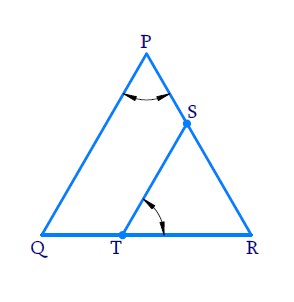### Solution

Reasoning:

If two angles of one triangle are respectively equal to two angles of another triangle, then the two triangles are similar.

This is referred as $$AA$$ criterion for two triangles.

Steps:

In $$\Delta RPQ,\,\,\Delta RTS$$

\begin{align} & \angle RPQ=\angle RTS\,\,\,\,( \text{Given} ) \\ & \angle PRQ=\angle TRS\\&( \text{Common Angle} ) \\\\ & \Rightarrow \Delta RPQ-\Delta RTS\\&( \because \, \text{AA Criterion}) \\ \end{align}

## Chapter 6 Ex.6.3 Question 6

In Figure, if $$\Delta \mathrm{ABE} \cong \Delta \mathrm{ACD}$$, show that $$\Delta ADE \sim \Delta ABC$$.

Diagram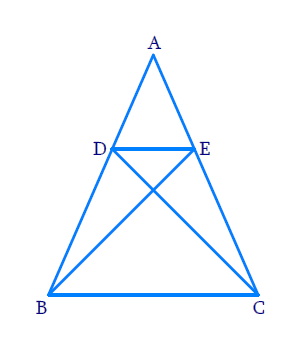### Solution

Reasoning:

As we know if two triangles are congruent to each other; their corresponding parts are equal.

If one angle of a triangle is equal to one angle of the other triangle and the sides including these angles are proportional, then the two triangles are similar.

This criterion is referred to as the SAS (Side–Angle–Side) similarity criterion for two triangles.

Steps:

In \begin{align}\Delta ABE\,\,{\rm {and}}\,\Delta ACD \end{align}

\begin{align} & AE=AD\\ [ \because \Delta ABE & \cong \Delta ACD \quad (\text{Given})]\ldots (1) \\\\ & AB=AC &\\ [ \because \Delta ABE &\cong \Delta ACD \quad (\text{Given} )]\ldots (2) \\ \end{align}

Now Consider $$\Delta ADE,\,\,\Delta ABC$$

\begin{align} \frac{AD}{AB}&=\frac{AE}{AC}\;\dots\text{from}\,\,(1)\,\And (2)\\\\&\rm{and} \\\\ \angle DAF&=\angle BAC \\text{Com}&\text{mon } \text{angle} ) \\\\ \Rightarrow \Delta ADE&\sim{\ }\Delta ABC\\ ( \text{SAS }&\text{criterion} ) \end{align} ## Chapter 6 Ex.6.3 Question 7 In Figure, altitudes \(AD and $$CE$$ of $$\Delta ABC$$ intersect each other at the point .Show that:

(i)  $$\Delta AEP\sim{\ }\Delta CDP$$

(ii)  $$\Delta ABD\sim{\ }\Delta CBE$$

(iii)  $$\Delta AEP\sim\Delta ADB$$

(iv)  $$\Delta \text{ }PDC\sim\Delta BEC$$

Diagram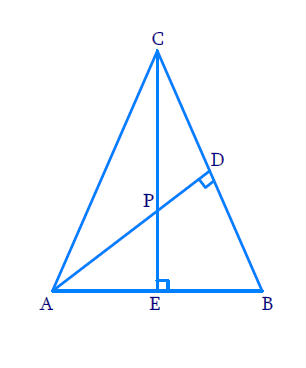### Solution

(i) Reasoning:

If two angles of one triangle are respectively equal to two angles of another triangle, then the two triangles are similar.

This may be referred to as the $$AA$$ similarity criterion for two triangles.

Steps:

In $$\Delta AEP$$ and $$\Delta CDP$$

\begin{align}&\angle AEP = \angle CDP = {90^\circ }\\&\left[ \begin{array}{l}\therefore CE \bot AB\,\,{\rm{and}}{\mkern 1mu} {\mkern 1mu} {\rm{AD}} \bot {\rm{BC}};{\mkern 1mu} \,{\rm{altitudes}}\end{array} \right]\\\\&\angle APE = \angle CPD\\&\;\;\left[ \begin{array}{l}{\rm{Vertically}}\,{\rm{opposite}}{\mkern 1mu} \,{\rm{angles}}\end{array} \right]\\\\& \Rightarrow \Delta AEP\sim\Delta CPD\\&\qquad\left[ {{\text{AA Criterion}}} \right]\end{align}

(ii) Reasoning:

If two angles of one triangle are respectively equal to two angles of another triangle, then the two triangles are similar.

This may be referred to as the $$AA$$ similarity criterion for two triangles.

Steps:

In $$\Delta ABD\,{\rm {and}}\,\Delta CBE$$

\begin{align}\angle ADB &= \angle CEB = {90^\circ }\\\angle ABD &= \angle CBE\\ &[ \text{Common angle}]\\\\ \Rightarrow \,\Delta ABD &\sim \Delta CBE\\ [AA&\text{ Criterion}]\end{align}

(iii) Reasoning:

If two angles of one triangle are respectively equal to two angles of another triangle, then the two triangles are similar.

This may be referred to as the $$AA$$ similarity criterion for two triangles.

Steps:

In $$\Delta\rm{ A E P}$$ and $$\Delta\rm{ A D B}$$

\begin{align}\angle AEP &= \angle ADB = {90^\circ }\\\angle PAE &\!=\! \angle BAD \\ &[ \text{Common Angle}]\\\\ \Rightarrow \quad \Delta AEP &\sim \Delta ADB\end{align}

(iv) Reasoning:

If two angles of one triangle are respectively equal to two angles of another triangle, then the two triangles are similar.

This may be referred to as the $$AA$$ similarity criterion for two triangles.

Steps:

In $$\Delta P D C \,{\rm{and}}\, \Delta B E C$$

\begin{align}\angle PDC &= \angle BEC = {90^\circ }\\\angle PCD &= \angle BCE \\& [\text{Common Angle}]\\\\ \Rightarrow \quad\Delta PDC &- \Delta BEC\end{align}

## Chapter 6 Ex.6.3 Question 8

$$E$$ is a point on the side $$AD$$ produced of a parallelogram $$ABCD$$ and $$BE$$ intersects $$CD$$ at $$F.$$ Show that $$\Delta ABE \sim \Delta CFB.$$

Diagram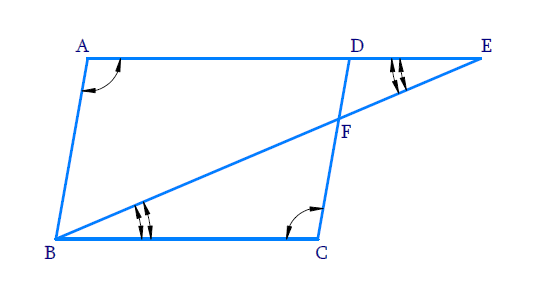### Solution

Reasoning:

If two angles of one triangle are respectively equal to two angles of another triangle, then the two triangles are similar.

This may be referred to as the $$AA$$ similarity criterion for two triangles.

Steps:

In $$\Delta \rm{A B E}, \Delta \rm{C F B}$$

$$\angle B A E=\angle F C B$$

$$\text{(opposite angles of a parallelogram)}$$

$$\angle A E B=\angle F B C$$

\begin{align} \begin{bmatrix} \because AE \,||\, BC \; \text{ and } \; EB \\ \text{ is a transversal alternate angle}\end{bmatrix} \end{align}

$$\Rightarrow\;\, \Delta ABE \sim \Delta C F E$$

$$\text{(AA Criterion)}$$

## Chapter 6 Ex.6.3 Question 9

In Figure, $$ABC$$ and $$AMP$$ are two right triangles, right angled at $$B$$ and $$M$$ respectively.

Prove that:

(i) \begin{align}\Delta ABC \sim \Delta AMP\end{align}

(ii) \begin{align}\frac{CA}{PA}=\frac{BC}{MP}\end{align}

Diagram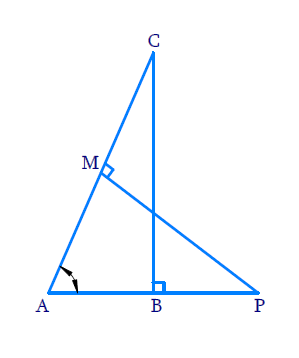### Solution

(i) Reasoning:

If two angles of one triangle are respectively equal to two angles of another triangle, then the two triangles are similar.

This may be referred to as the $$AA$$ similarity criterion for two triangles.

Steps:

In $$\Delta {ABC}$$ and $$\Delta {AMP}$$

\begin{align} & \angle ABC=\angle AMP={{90}^{^\circ }} \\& \,\angle BAC\!=\!\angle MAP\\& \qquad ( \text{Common angle}) \\\\& \;\;\Delta ABC\sim\Delta AMP \\\end{align}

(ii) Reasoning:

As we know that the ratio of any two corresponding sides in two equiangular triangles is always the same

Steps:

In $$\Delta {ABC}$$  and  $$\Delta {AMP}$$

\begin{align}\frac{CA}{PA}&=\frac{BC}{MP}\\because \Delta ABC &\sim \Delta AMP)\end{align} ## Chapter 6 Ex.6.3 Question 10 \(CD and $$GH$$ are respectively the bisectors of $$\angle ACB$$ and $$\angle EGF$$ such that $$D$$ and $$H$$ lie on sides $$AB$$ and $$FE$$ of $$\Delta ABC$$ and $$\Delta EFG$$ respectively. If $$\Delta ABC \sim \Delta FEG$$, show that:

(i)\begin{align}\frac{CD}{GH}=\frac{AC}{FG}\end{align}

(ii)\begin{align}\text{ }\Delta DCB\text{ }\sim{\ }\text{ }\Delta HGE\end{align}

(iii) \begin{align}\Delta DCA\text{ }\sim{\ }\Delta HGF\end{align}

Diagram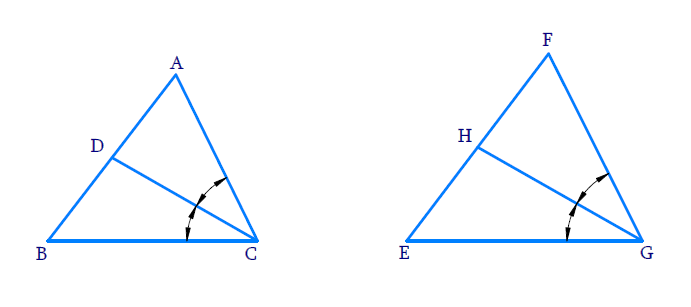### Solution

(i) Reasoning:

If two angles of one triangle are respectively equal to two angles of another triangle, then the two triangles are similar.

This may be referred to as the $$AA$$ similarity criterion for two triangles.

Steps:

$$\angle ACB\,=\,\angle FGE$$

\begin{align} \Rightarrow \frac {{\angle ACB}}{2}= \frac {{\angle FGE}}{2} \end{align}

$$\Rightarrow \angle ACD\,=\,\angle FGH$$ ($$CD$$ and $$GH$$ are bisectors of $$\angle C {\,\rm{and}} \,\angle G$$ respectively)

In $$\Delta ADC$$ and $$\Delta FHG$$

\begin{align}&\angle D A C=\angle H F G\\&[\because \Delta A D C \sim \Delta F E G]\\\\&\angle A C D=\angle F G H\\\Rightarrow \;&\Delta A D C\,\sim\,\Delta F H G\\& \;\;\text{(AA Criterion)} \end{align}

If two triangles are similar, then their corresponding sides are in the same ratio.

\begin{align} \Rightarrow \frac{C D}{G H}=\frac{A C}{F G}\end{align}

(ii) Reasoning:

If two angles of one triangle are respectively equal to two angles of another triangle,then the two triangles are similar.

This is reffered as AA criterion for two triangles.

Steps:

In $$\Delta DCB$$ and $$\Delta HGE$$

\begin{align}&\angle DBC=\angle HEG\,\\&\left[\because \Delta ABC\sim \Delta FEG \right] \\\\ &\angle DCB=\angle HGE\\&\left[\because \frac{\angle ACB}{2}=\frac{\angle FGE}{2} \right] \\\\\Rightarrow&\quad \Delta DCB \sim \Delta EHG\\&\quad\text{(AA Criterion}) \\ \end{align}

(iii) Reasoning:

If two angles of one triangle are respectively equal to two angles of another triangle,then the two triangles are similar.

This is reffered as AA criterion for two triangles.

Steps:

In $$\Delta DCA,\,\,\Delta HGF$$

\begin{align}& \angle DAC=\angle HFG\\&\left[ \because\Delta ABC\sim \Delta FEG \right] \\\\ & \angle ACD=\angle FGH\\&\left[\because \frac{{\angle ACB}}{2} = \frac {{\angle FGE}}{2}\right] \\\\ \Rightarrow\quad &\Delta DCA\sim \Delta HGF\\&\;\;\text{(AA Criterion}) \\ \end{align}

## Chapter 6 Ex.6.3 Question 11

In Figure $$, E$$ is a point on side $$CB$$ produced of an isosceles $$\triangle ABC$$ with $$AB = AC$$. If $$AD \bot BC$$ and $$EF \bot AC$$, prove that $$\Delta A B D \sim \Delta E C F$$.

Diagram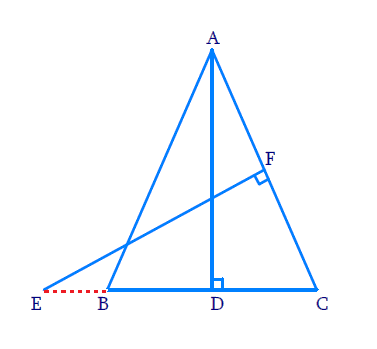### Solution

Reasoning:

If two angles of one triangle are respectively equal to two angles of another triangle, then the two triangles are similar.

This is referred as the $$AA$$ criterion for two triangles.

Steps:

In $$\Delta ABD,\,\,\Delta ECF$$

\begin{align}&\angle ADB=\angle EFC={{90}^{\circ }}\\& (\because AD\bot BC \text{and}\,EF\bot AC) \\ &\angle ABD=\angle ECF \\& \begin{bmatrix}\because \text{In}\,\Delta ABC,AB=AC\\\Rightarrow \angle ABC=\angle ACB\end{bmatrix} \\&\Rightarrow \Delta ABD \sim \Delta ECF\\&\qquad(\text{AA}\,\,\text{criterion}) \\ \end{align}

## Chapter 6 Ex.6.3 Question 12

Sides $$AB$$ and $$BC$$ and median $$AD$$ of a $$\triangle ABC$$ are respectively proportional to sides $$PQ , QR$$ and median $$PM$$ of $$\Delta PQR$$ (see the below Figure) .

Show that $$\Delta ABC$$~ $$\Delta PQR$$.

Diagram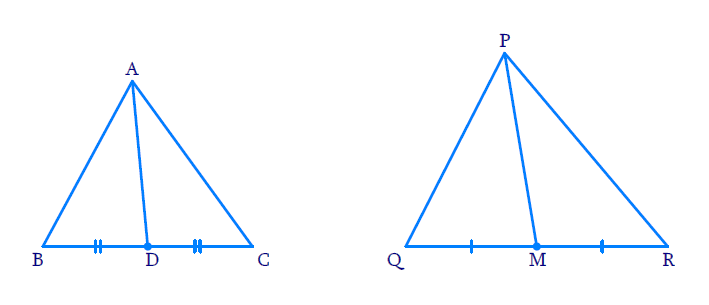### Solution

Reasoning:

As we know if one angle of a triangle is equal to one angle of the other triangle and the sides including these angles are proportional, then the two triangles are similar.

This is referred as $$SAS$$ (Side–Angle–Side)  criterion for two triangles.

Steps:

In $$\Delta ABC$$ and $$\Delta PQR$$

\begin{align} \frac{AB}{PQ}=\frac{BC}{QM}=\frac{AD}{PM} \,\,\,\text{[Given]} \\ \end{align}

$$\because$$ AD  and PM are median of $$\Delta ABC$$ and $$\Delta PQR$$ respectively

\begin{align}&\Rightarrow \frac{BD}{QM}=\frac{{\frac{{BC}}{2}}}{{\frac{{QR}}{2}}}={\frac{BC}{QR}} \end{align}

Now In $$\Delta \rm{ABD}\,\,\text {and}\,\Delta \rm{PQM}$$

\begin{align}&\frac{AB}{PQ}=\frac{BD}{QM}=\frac{AD}{PM} \\ \end{align}

$\Rightarrow \Delta \rm{A B D} \sim \Delta \rm{P Q M}$

Now in $$\Delta \rm{A B C}\,\text{and}\, \Delta \rm{P Q R}$$

\begin{align}&\frac{A B}{P Q}=\frac{B C}{Q R}\\&(\text {Given in the statement}) \\ &\angle A B C=\angle P Q R\\&[\because \Delta A B D\sim\Delta P Q M] \\ \Rightarrow\! \Delta A B C &\!\sim \! \Delta P Q R \,\,[\text{SAS} \text { Criterion }\!\!]\end{align}

## Chapter 6 Ex.6.3 Question 13

$$D$$ is a point on the side $$BC$$ of a $$\Delta ABC$$ such that $$\angle ADC$$ $$=$$ $$\angle BAC$$. Show that $$\,C{{A}^{2}}=CB.CD$$ .

Diagram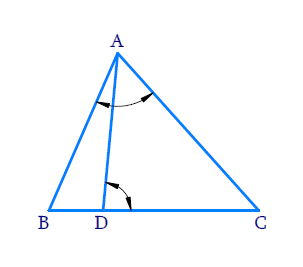### Solution

Reasoning:

As we know if two triangles are similar then their corresponding sides are proportional.

Steps:

In $$\Delta ABC$$ and $$\Delta DAC$$

\begin{align} & \angle BAC=\angle ADC \\ & \text{(Given in the statement)}\\\\ & \angle ACB=\angle ACD\\ & \text{(Common angles)} \\\\ \Rightarrow \quad&\Delta ABC \sim \Delta DAC \\ & \text{(AA criterion)} \end{align}

If two triangles are similar,then their corresponding sides are proportional

\begin{align} \Rightarrow \quad\frac{C A}{C D}&=\frac{C B}{C A}\\\Rightarrow \quad C A^{2}&=C B \cdot C D\end{align}

## Chapter 6 Ex.6.3 Question 14

Sides $$AB$$ and $$AC$$ and median $$AD$$ of a $$\Delta ABC$$ are respectively proportional to sides $$PQ$$ and $$PR$$ and median $$PM$$ of another $$\Delta PQR.$$

Show that $$\Delta ABC\text{ }\sim{\ }\text{ }\Delta PQR$$ .

Diagram### Solution

Reasoning:

As we know if one angle of a triangle is equal to one angle of the other triangle and the sides including these angles are proportional, then the two triangles are similar.

This criterion is referred to as the $$\rm SAS$$ (Side–Angle–Side) similarity criterion for two triangles.

Steps: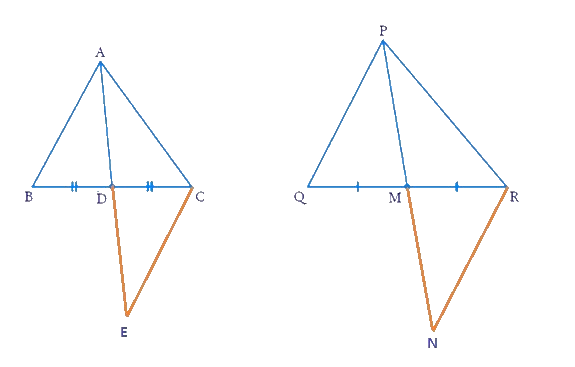Produce $$AD$$  to $$E$$ so that $$AD = DE.$$ Join $$CE$$ Similarly, produce $$PM$$ to $$N$$ such that $$PM = MN$$, and Join $$RN$$.

In $$\Delta ABD$$  and $$\Delta CDE$$

\begin{align}&\quad \;\, AD = DE\\ \text{[By } & \text{Construction]}\\\\&\quad \;\, BD = DC\\ [\text{AP } & \text{is the median}] \\\\& \angle ADB = \angle CDE \\ [ \text{Vertically }&\text{opposite angles}] \\\\\therefore &\Delta ABD \cong \Delta CDE \\ [\text{By SAS } & \text{Criterion of congruence}] \\\\ \Rightarrow &\quad \;\; AB = CE \;[ CPCT] \dots \text{(i)} \end{align}

Also, in $$\Delta PQM$$ and $$\Delta MNR$$

\begin{align}PM &= MN \\ \text{[By }&\text{Construction]}\\\\ QM &= MR \\ \text{[PM is } &\text{the median]} \\\\ \angle PMQ &= \angle NMR \\ \text{[Vertically }& \text{opposite angles]} \\\\ \therefore \Delta PQM &= \Delta MNR \\ \text{By SAS }& \text{criterion of congruence}\\\\ \Rightarrow PQ &= RN [CPCT] \text{... (ii)}\end{align}

Now,

\begin{align} &\frac{{AB}}{{PQ}} = \frac{{AC}}{{PR}} = \frac{{AD}}{{PM}} \;\; \left[ {{\rm{Given}}} \right]\\ &\Rightarrow \frac{{CE}}{{RN}} = \frac{{AC}}{{PR}} = \frac{{AD}}{{PM}}\\ &\qquad [\text{From (i)} \text{ and (ii)} ] \\\\& \Rightarrow \frac{{CE}}{{RN}} = \frac{{AC}}{{PR}} = \frac{{2AD}}{{2PM}}\\ &\Rightarrow \frac{{CE}}{{RN}} = \frac{{AC}}{{PR}} =\frac{{AE}}{{PN}} \\ &[ 2AD = AE\;{\text{ and }}\; 2PM = PN ] \\\\ &\therefore \Delta ACE\sim\Delta PRN \\& [ \text{By SSS} \text{ Similarity} \text{ Criterion}]\end{align}

Therefore,

$\angle CAE = \angle RPN$

Similarly,

$\angle BAE = \angle QPN$

Therefore,

\begin{align} \angle CAE \!\!+\! \angle BAE &\!=\! \angle RPN \!\! +\!\! \angle QPN \\ \Rightarrow \angle BAC &= \angle QPR\\ \Rightarrow \angle A &= \angle P....{\rm{(iii)}}\end{align}

Now, in \begin{align}\Delta ABC\;{\text{ and }}\;\Delta PQR\end{align}

\begin{align}&\frac{{AB}}{{PQ}} = \frac{{AC}}{{PR}}\\&\;\;\angle A = \angle P\quad \dots\left[ {{\rm{from (iii)}}} \right]\\\\&\therefore \Delta ABC\sim\Delta PQR \\ & [ \text{By SAS} \text{ similarity} \text{ criterion} ] \end{align}

## Chapter 6 Ex.6.3 Question 15

A vertical pole of length $$6$$ $$\rm m$$ casts a shadow $$4$$ $$\rm m$$ long on the ground and at the same time a tower casts a shadow $$28$$ $$\rm m$$ long. Find the height of the tower.

Diagram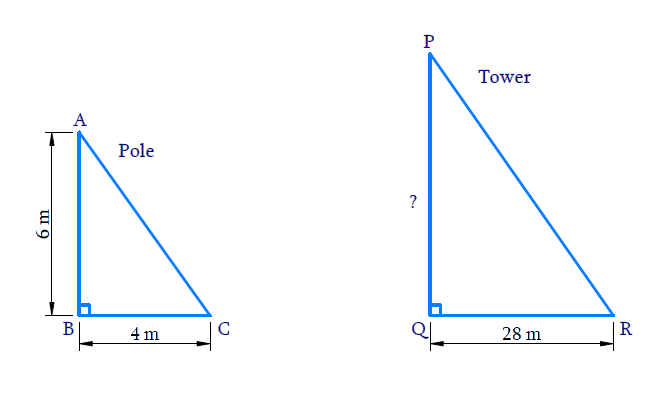### Solution

Reasoning:

The ratio of any two corresponding sides in two equiangular triangles is always the same.

Steps:

$$AB$$ is the pole $$=$$ $$6$$ $$\rm m$$

$$BC$$ is the shadow of pole $$=$$ $$4$$ $$\rm m$$

$$PQ$$ is the tower $$=?$$

$$QR$$ is the shadow of the tower $$=$$ $$28$$ $$\rm m$$

In $$\Delta ABD$$ and $$\Delta PQR$$

\begin{align}&\angle ABC=\angle PQR= 90^{\circ} \\ &\begin{bmatrix}\text{The objects and shadow are } \\ \text{perpendicular to each other}\end{bmatrix}\end{align}

\begin{align}&\angle BAC=\angle QPR \\ &\begin{bmatrix} \text{Sunrays fall on the }\\\text{pole and tower at the }\\ \text{same angle, at the same time} \end{bmatrix} \end{align}

\begin{align}\Rightarrow \quad \Delta ABC \sim \Delta PQR \\ \text{(AA Criterion)} \end{align}

The ratio of any two corresponding sides in two equiangular triangles is always the same.

\begin{align} \Rightarrow\qquad \frac{A B}{B C}&=\frac{P Q}{Q R} \\ \frac{6 \rm {}m}{6 \rm{}m}&=\frac{P Q}{28\rm{} m} \\ \Rightarrow\quad P Q&=\frac{6 \times 28}{4}{\rm {m}} \\ P Q &=42\rm{} m \end{align}

Hence, the height of the tower is $$42 \rm{}\,m.$$

## Chapter 6 Ex.6.3 Question 16

If AD and PM are medians of triangles ABC and PQR, respectively where $$\Delta ABC\sim\Delta PQR$$, prove that \begin{align}\frac{AB}{PQ}=\frac{AD}{PM} \end{align}·

Diagram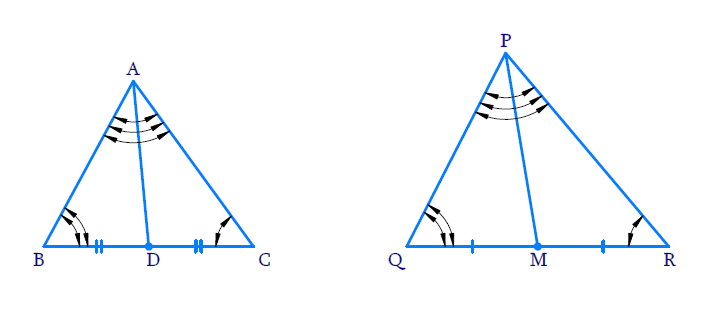### Solution

#### Reasoning:

As we know if one angle of a triangle is equal to one angle of the other triangle and the sides including these angles are proportional, then the two triangles are similar.

This is referred as SAS (Side–Angle–Side) Criterion for two triangles.

#### Steps:

\begin{align}&\Delta A B C \sim \Delta P Q R\\\\\Rightarrow \quad&\angle A B C=\angle P Q R \\ &\text{(corresponding angles)} \dots(1)\\\\ \Rightarrow \quad &\;\;\;\,\frac{A B}{P Q}=\frac{B C}{Q R} \\& \text{(corresponding sides)} \\\\\Rightarrow \quad & \;\;\;\,\frac{A B}{P Q}=\frac{B C / 2}{Q R / 2} \\\\\Rightarrow \quad & \;\;\;\,\frac{AB}{PQ}=\frac{BD}{QM} \\ & \begin{bmatrix}D\text{ and }M \text{are mid points } \\ \text{of } BC \text{ and }QR\end{bmatrix} \dots(2) \end{align}

In $$\Delta ABD,\Delta PQM$$

\begin{align}\angle A B D=\angle P Q M\;\;\dots \text{(from 1)}\end{align}

\begin{align}\frac{A B}{P Q}=\frac{B D}{Q M}\;\;\dots \text{(from 2)}\end{align}

\begin{align}\Rightarrow \quad&\Delta A B D \sim \Delta P Q M \\& \text{(SAS Criterion)}\end{align}

\begin{align}\Rightarrow \quad & \frac{A B}{P Q}=\frac{B D}{Q M}=\frac{A D}{P M}\\& \text{(Corresponding sides)}\end{align}

\begin{align} \Rightarrow \frac{A B}{P Q}&=\frac{A D}{P M}\end{align}

Download SOLVED Practice Questions of NCERT Solutions For Class 10 Maths Chapter 6 Exercise 6.3 for FREE
Ncert Class 10 Exercise 6.3
Ncert Solutions For Class 10 Maths Chapter 6 Exercise 6.3
Instant doubt clearing with Cuemath Advanced Math Program